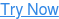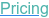#### SOFTWARE#### KNOWLEDGE BASE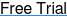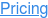# Sample Size for Categorical Endpoints

March 7, 2023

Categorical data is among the most common types of data found in clinical trials and comes in a variety of different forms such as binary and ordered categorical data.

In this webinar, we explore the different types of categorical data found in research, and then how to select the statistical method and sample size calculation most appropriate for each specific type of categorical endpoint and design.

## Sample Size for Categorical EndpointsBinary, Ordered & Contingency Table Data### In this free webinaryou will learn about:

• Types of Categorical Data

• Statistical Methods for Categorical Data

• Sample Size for Categorical Data Methods

Categorical (also known as proportion) data is among the most common types of data found in clinical trials. In wider scientific research, three common types are binary data, ordered categorical data and contingency table data.

In this webinar, we examine some practical problems you are likely to encounter and offer solutions using the following methods:

1. Sample Size for Two Independent Proportions (Chi-Squared, Exact Tests)
2. Sample Size for Two Ordered Categorical (Proportional Odds Model)
3. Power for Chi-squared Independence Test for 2-Way Contingency Table

Binary Data is data where subjects are categorized into one of two categories (e.g. disease/no disease) as the outcome of interest. This data is extremely common across various clinical areas with common statistical methods for binary data including chi-squared tests and logistic regression.

Ordered categorical (also known as ordinal) data is where subjects are categorized into one of the available categories and in which each category is ordered from best to worst. Scales for areas such as pain or disability are a common example in clinical trials with common statistical methods including the proportional odds model and the Wilcoxon test.

Contingency table data (also known as cross-tab) shows the frequency between and within two or more categorical outcomes and would be common in areas such as epidemiology. Tests for independence such as the chi-squared and Fisher’s exact tests are commonly used for this type of data.

So join us for this webinar as we explore the types of categorical data, the different designs and statistical methods appropriate for each type, and the sample size determination methods available for each of these contexts.

### Play the video below to watchthe complete recording of this webinar

Duration: 60 minutes

 Explore The Data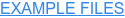Get The Deck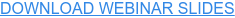• Contingency Chi-squared nQuery Example
• Fishers Test nQuery Example
• Proportional Odds Model nQuery Example
• Wilcoxon nQuery Example

Are you looking for more trial design resources
Webinars to improve clinical trial designs & Practical examples of sample size determination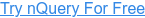## You May Also Like

These Stories on Guide to Sample Size